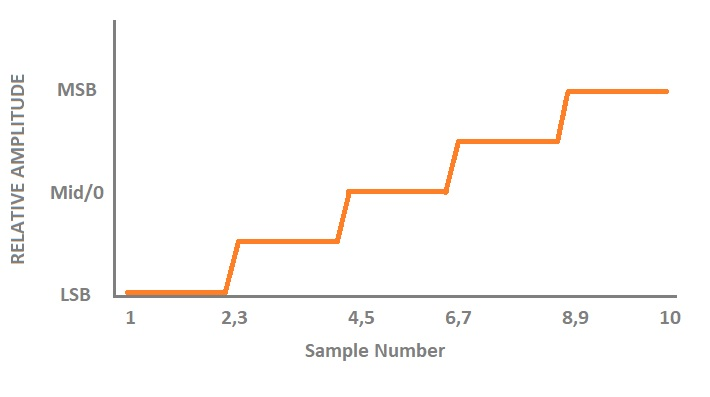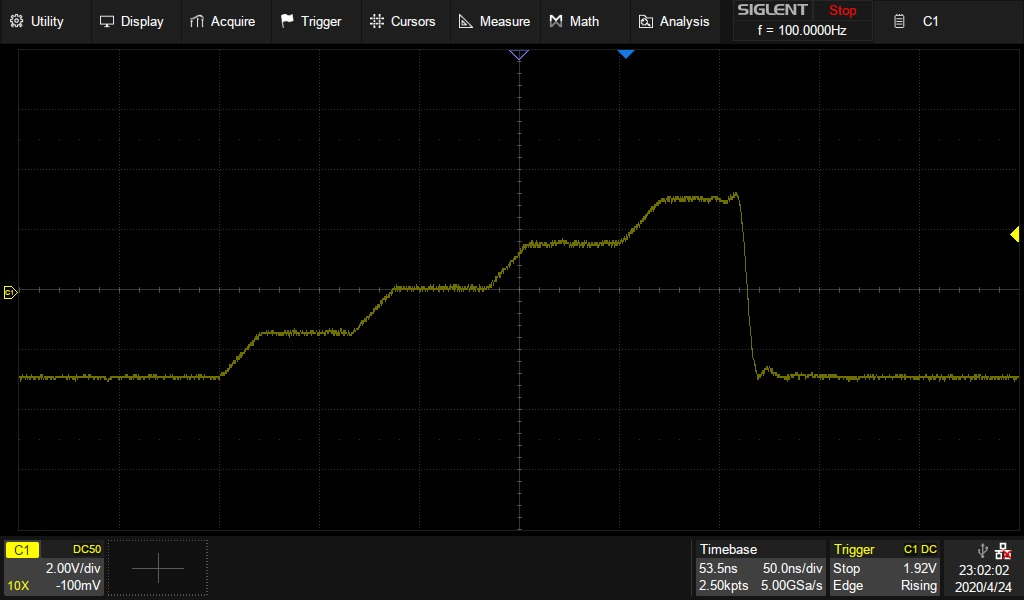# Programming Example: Create a stair-step waveform using Python and PyVISA using LAN (SDG1000X, SDG2000X, SDG6000X)

April 24, 2020

The SIGLENT SDG series of arbitrary waveform generators can use waveforms created programmatically.

The waveform data can be sent as individual samples formatted as binary little-endian, 2s complement values.

In this programming example, we create a 10 point arbitrary waveform that starts at the least-significant bit and steps up to the most-significant bit to help with understanding the required sample format as well as provide a base for your own waveform creation.

Here is a picture of the desired waveform:The SDG waveform data requires each sample to first be formatted as binary, little-endian, 2’s complement. For easier human viewing, the example enters the data in hex format and then “unhexes” the data before it is added to the command string which is then sent to the instrument.

Here is a table showing the value of each sample and the value in hex for 14-bit (SDG1000X series) and 16-bit (SDG2000X/SDG6000X) instruments:Here is an oscilloscope capture of a single burst of this waveform:Here is a link to a Zipped version of the .PY file: SDG PyVISA LAN Arb

Here is the text of the program:

```#SDG PyVISA LAN Arb
#Creates a 10 point stepped arbitrary waveform.
#The step starts with least significant bit (LSB) x 2, (Middle bit - LSB)/2 bit x 2, middle bit x 2,
#Most significant bit (MSB - Middle Bit)/2 x 2, and MSB x 2
#
#Sends using PyVISA LAN connection
#
#Author: JAC
#Date: 04/24/2020
#
#!/usr/bin/env python3
# -*- coding: utf-8 -*-

import visa
import time
import binascii
import random

#VISA resource of Device
device_resource = 'TCPIP0::192.168.0.19::inst0::INSTR'

#Little endian, 16-bit 2's complement

#send 10 points to the generator.
#16 bit for SDG6000X and SDG2000X
#hex(-32768)= 0X8000, hex(-16384)=0XC000, hex(0)=0X0000, hex(16384)=0X4000, hex(32768)=0X8000
#wave_data = [0x8000, 0x8000, 0xc000, 0xc000, 0x0000, 0x0000, 0x4000, 0x4000, 0x8000, 0x8000]
#
#14 bit data for SDG1000X
#hex(-8192)= 0XE000, hex(-4096)=0XF000, hex(0)=0X0000, hex(4096)=0X1000, hex(8192)=0X2000
wave_data = [0xe000, 0xe000, 0xf000, 0xf000, 0x0000, 0x0000, 0x1000, 0x1000, 0x2000, 0x2000]
wave_points = []

for i in range(0,1):
wave_points += wave_data
count=1

print(wave_points)
print(len(wave_points))

def create_wave_file():
"""create a file"""
f = open("wave1.bin", "wb")
for a in wave_points:
b = hex(a)
b = b[2:]
len_b = len(b)
if (0 == len_b):
b = '0000'
elif (1 == len_b):
b = '000' + b
elif (2 == len_b):
b = '00' + b
elif (3 == len_b):
b = '0' + b
b = b[2:4] + b[:2] #change big-endian to little-endian
c = binascii.unhexlify(b)    #Hexadecimal integer to ASCii encoded string
f.write(c)
f.close()

def send_wave_data(dev):
"""send wave1.bin to the device"""
f = open("wave1.bin", "rb")    #wave1.bin is the waveform to be sent
print('write class:', type(data))
print('write bytes:',len(data))
dev.write_termination = ''
dev.write("C1:WVDT WVNM,wave1,FREQ,2000.0,AMPL,4.0,OFST,0.0,PHASE,0.0,WAVEDATA,%s"%(data),encoding='latin1')    #"X" series (SDG1000X/SDG2000X/SDG6000X/X-E)&amp;amp;amp;lt;/pre&amp;amp;amp;gt;
dev.write("C1:ARWV NAME,wave1")
f.close()
return data

if __name__ == '__main__':
""""""
#device = visa.instrument(device_resource, timeout=30*60*1000, chunk_size = 16*1024*1025)
rm=visa.ResourceManager()
device=rm.open_resource(device_resource, timeout=50000, chunk_size = 24*1024*1024)
create_wave_file()
send=send_wave_data(device)

```